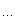# woaidongmao

## Trait和Template Mataprogram的奥秘

C++是一门复杂的语言，之所以复杂，就是因为被很多的大牛们发掘出了它的很多极其怪异的用法，或者也可以说是很高明的技巧，这些技巧，我们普通人难以掌握，也很少有非使用不可的时候，但是对于那些大牛们来说，却是必不可少的利器。不信的话，翻翻Boost的源码，这样复杂的技巧比比皆是。

template <

class CharType,

class Traits=char_traits<CharType>,

class Allocator=allocator<CharType>
>
class basic_stringtypedef basic_string<char> stringbasic_string< char, char_traits<char>, allocator<char> >

class CMyWindow: public CWindowImpl<
CMyWindow,
CWindow,
CWinTraits
<WS_OVERLAPPEDWINDOW|WS_VISIBLE,0>
>
{};

template <typename T>
T average(T
const* begin, T const* end)
{
T total
= T();

int count = 0;

while (begin != end){
total
+= * begin;

++begin;

++count;
}

}

int main(){

int numbers[] = {1,2,3,4,5};
std::cout
<< average(&numbers,&numbers) << std::endl;
}

int main(){

char characters[] = "traits";
std::cout
<< static_cast<int>(average(&characters,&characters)) << std::endl;
}

template <typename T>
class TypeTraits;

template
<>
class TypeTraits<char>{
public:
typedef
int ReturnType;
}

template
<>
class TypeTraits<short>{
public:
typedef
int ReturnType;
}

template
<>
class TypeTraits<int>{
public:
typedef
int ReturnType;
}

template
<>
class TypeTraits<float>{
public:
typedef
double ReturnType;
}

template <typename T,typename Traits>
typename Traits::ReturnType average(T
const* begin, T const* end)
{
typedef typename Traits::ReturnType ReturnType;
ReturnType total
= ReturnType();

int count = 0;

while (begin != end){
total
+= * begin;

++begin;

++count;
}

}

int main(){

int numbers[] = {1,2,3,4,5};
std::cout
<< average<int,TypeTraits<int> >(&numbers,&numbers) << std::endl;

char characters[] = "traits";
std::cout
<< average<char,TypeTraits<char> >(&characters,&characters) << std::endl;
}

1、它编的程序不是运行的时候执行的，而是在编译的时候由编译器执行的；
2、它能够牵着编译器的鼻子走，靠的完全是符合标准的模板语法，不需要使用编译器的任何API；
3、它居然是图灵完备的，也就是说它什么事都能干。

template <int N>
class Factorial
{
public:

enum { result = N * Factorial<N-1>::result };
};

template<>
class Factorial<1>
{
public:

enum { result = 1 };
}

int main()
{
std::cout
<< Factorial<10>::result << std::endl;

return 0;
}

OK，事情就这么简单。大家都知道，递归可以代替循环，就只是对内存的消耗大一些，所以递归的层次不能太多。解决了循环的问题，那么分支结构如何解决呢？

template <bool C, typename Ta, typename Tb>
class IfThenElse;

template
<typename Ta, typename Tb>
class IfThenElse<true, Ta, Tb>{
public:
typedef Ta ResultT;
};

template
<typename Ta, typename Tb>
class IfThenElse<false, Ta, Tb>{
public:
typedef Tb ResultT;
};

posted on 2008-08-31 21:23 肥仔 阅读(359) 评论(0)  编辑 收藏 引用 所属分类: C++ 模板